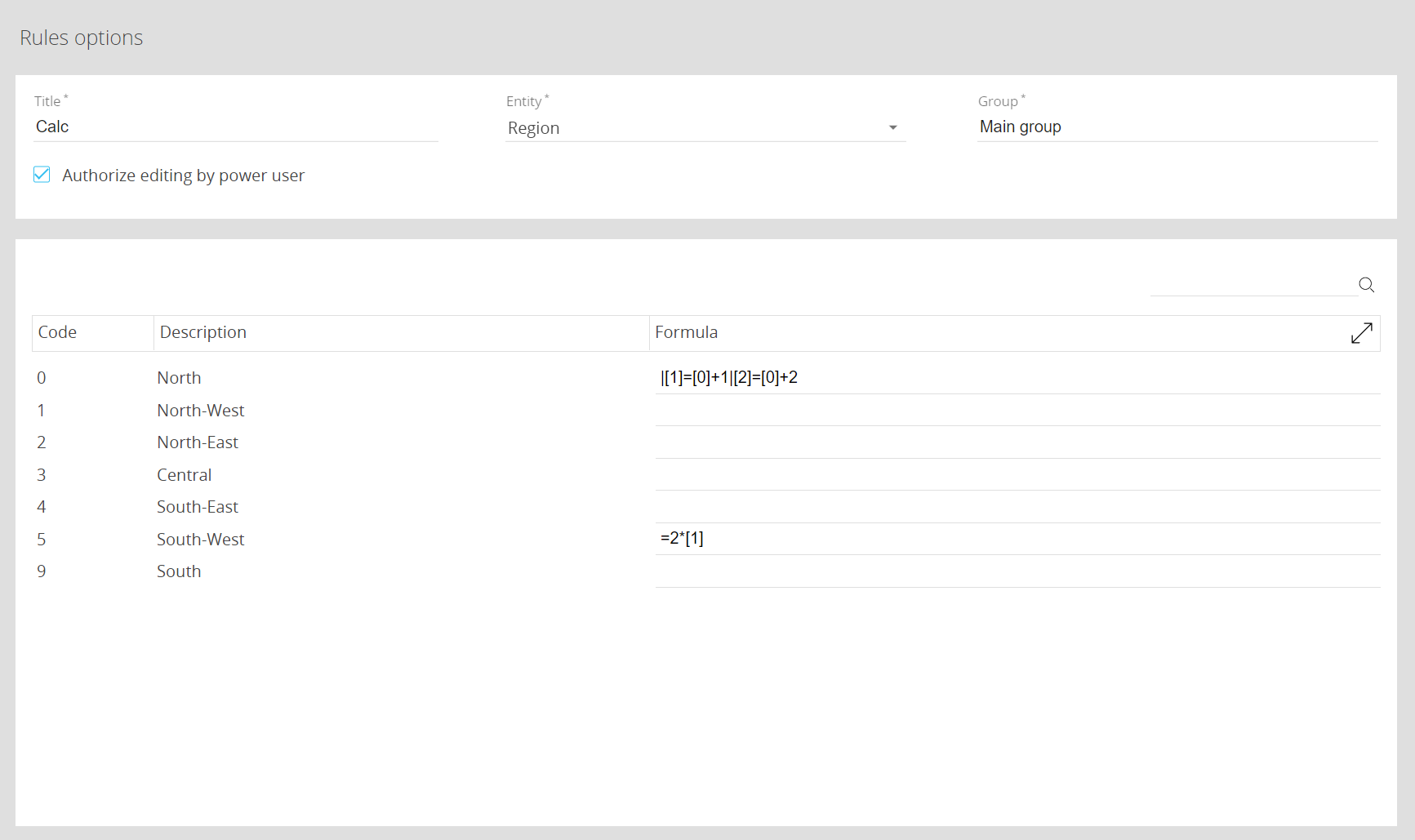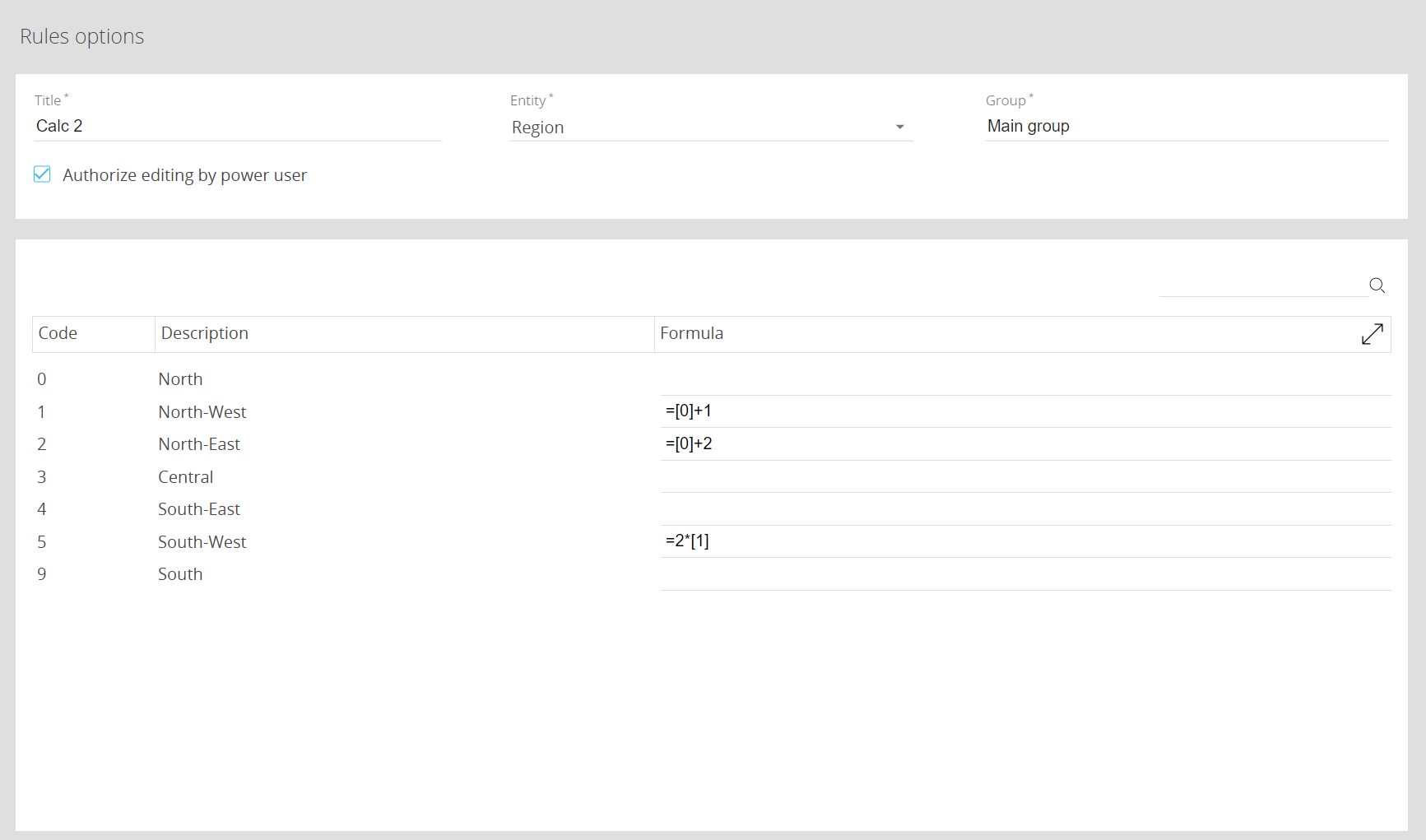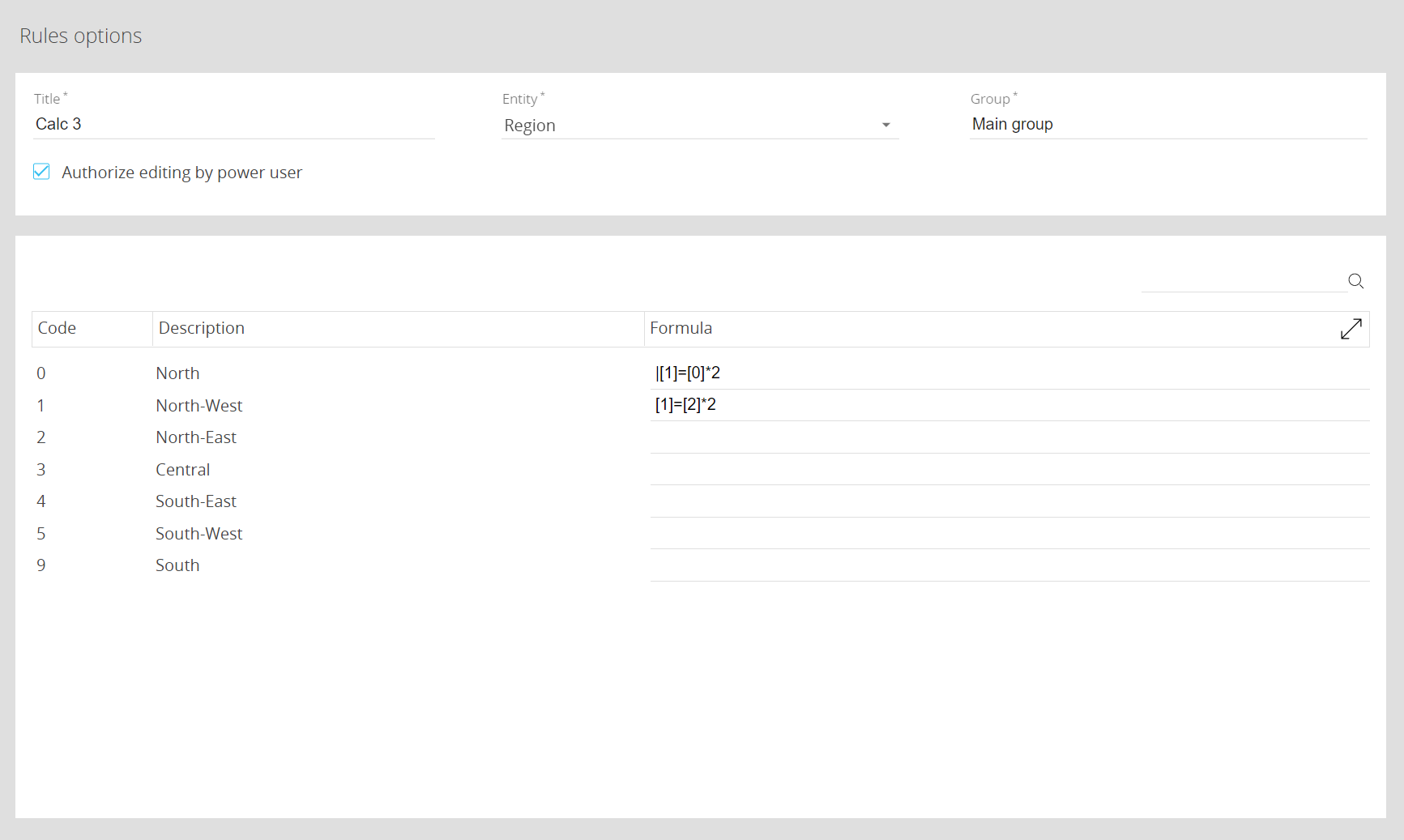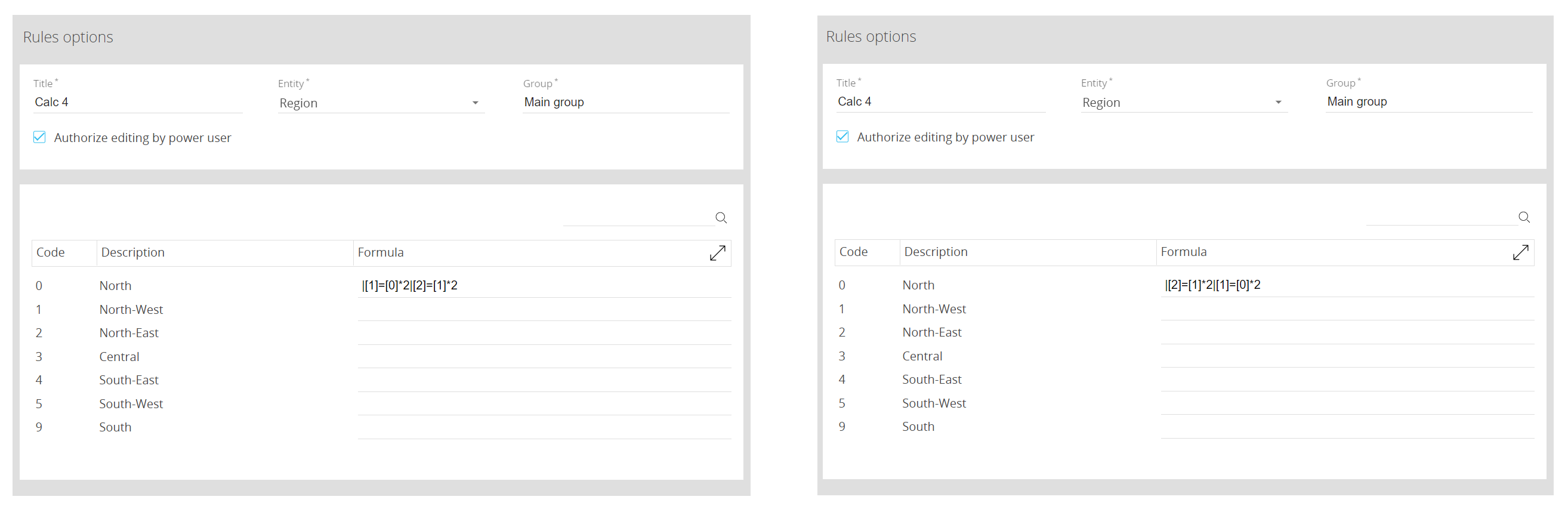# Reverse Rules and Data entry simulation

This topic describes how to create a reverse Rule and its specific properties.

A reverse Rule is a Rule with a specific syntax that allows it to write data to one or more Cube cells for other Entity members, depending on the value of the member where the reverse Rule has been defined.

## Defining a reverse Rule - Difference with a simple Rule

A reverse Rule is marked by a pipe character ("|") ahead of the formula. Reverse Rules can coexist with simple Rules in the same formula defined on a single member.

Let's consider the following scenario:In this scenario, as you enter any value for member  also members , , and  are calculated and written in the Cube, just like with a Data entry action: their value is the result of +1, +2, and 2*(+1) respectively.

The same result can also be obtained using the following simple Rule. The difference from the scenario shown above is that values for members  and  are not written in the Cube. In both scenarios, the value for member  is calculated and visualized, but not written in the Cube.You can set an unlimited number of reverse formulas for a single member.
You can set reverse Rules in combination with simple Rules in the same formula field, but simple Rules must always precede reverse Rules.
The result of the Rule calculation is immediately visible in the Layout preview as soon as the Rules is applied to a Cube.

## Simple Rules prevail on Reverse Rules

Let's consider the following scenario:In this case, the value saved for member  is equal to the double of the value for member , but the value visualized for member  is equal to the double of the value for member : there could be a misalignment between the written value and value visualized in reports.

## The order of formulas in a reverse Rule affect the results of the calculation

Reverse Rules are executed from left to right. For example, the following two Rules give different results:In the first Rule, if the value entered for member "0" is 10, then the value written for member "1" would be 20, and the value written for member "2" would be 40.

In the second Rule, if the value entered for member "0" is 10, then the value written for member "1" would be 0, and the value written for member "2" would be 20. This is because the first formula is executed before the second one, and the second one is the one that writes one of the arguments of the first formula.

The result of the reverse Rule calculation is immediately visible in the Screen as soon as a value is saved in the Cube. If Save/Undo mode is enabled on the Screen, after you have entered a value you have to click the save icon at the top left of the Data View to actually save the value in the Cube and trigger the Rule calculation.

## Known limitations

• Reverse Rules will not work if additional Entities are set By Row in the Layout, along with the Entity on which the reverse Rule has been defined
• For reverse Rules to work, the "Show all" property must be enabled for the By Row field in the Layout
• Reverse Rules will not work if the Entity on which the reverse Rule has been defined is set By Column
• The "Cube cells locked by" option is ignored if a Reverse Rule is defined to write data to a locked cell, since Reverse Rules are always allowed to write to any cell of the Cube.